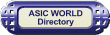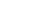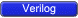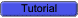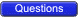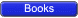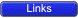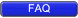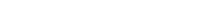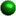Test Benches Ok, we have code written according to the design document, now what?Well we need to test it to see if it works according to specs. Most of the time, it's the same we use to do in digital labs in college days: drive the inputs, match the outputs with expected values. Let's look at the arbiter testbench.``` 1 module arbiter ( 2 clock, 3 reset, 4 req_0, 5 req_1, 6 gnt_0, 7 gnt_1 8 ); 9 10 input clock, reset, req_0, req_1; 11 output gnt_0, gnt_1; 12 13 reg gnt_0, gnt_1; 14 15 always @ (posedge clock or posedge reset) 16 if (reset) begin 17 gnt_0 <= 0; 18 gnt_1 <= 0; 19 end else if (req_0) begin 20 gnt_0 <= 1; 21 gnt_1 <= 0; 22 end else if (req_1) begin 23 gnt_0 <= 0; 24 gnt_1 <= 1; 25 end 26 27 endmodule 28 // Testbench Code Goes here 29 module arbiter_tb; 30 31 reg clock, reset, req0,req1; 32 wire gnt0,gnt1; 33 34 initial begin 35 \$monitor ("req0=%b,req1=%b,gnt0=%b,gnt1=%b", req0,req1,gnt0,gnt1); 36 clock = 0; 37 reset = 0; 38 req0 = 0; 39 req1 = 0; 40 #5 reset = 1; 41 #15 reset = 0; 42 #10 req0 = 1; 43 #10 req0 = 0; 44 #10 req1 = 1; 45 #10 req1 = 0; 46 #10 {req0,req1} = 2'b11; 47 #10 {req0,req1} = 2'b00; 48 #10 \$finish; 49 end 50 51 always begin 52 #5 clock = ! clock; 53 end 54 55 arbiter U0 ( 56 .clock (clock), 57 .reset (reset), 58 .req_0 (req0), 59 .req_1 (req1), 60 .gnt_0 (gnt0), 61 .gnt_1 (gnt1) 62 ); 63 64 endmodule ``` You could download file arbiter.v hereIt looks like we have declared all the arbiter inputs as reg and outputs as wire; well, that's true. We are doing this as test bench needs to drive inputs and needs to monitor outputs.After we have declared all needed variables, we initialize all the inputs to known state: we do that in the initial block. After initialization, we assert/de-assert reset, req0, req1 in the sequence we want to test the arbiter. Clock is generated with an always block.After we are done with the testing, we need to stop the simulator. Well, we use \$finish to terminate simulation. \$monitor is used to monitor the changes in the signal list and print them in the format we want.``` req0=0,req1=0,gnt0=x,gnt1=x req0=0,req1=0,gnt0=0,gnt1=0 req0=1,req1=0,gnt0=0,gnt1=0 req0=1,req1=0,gnt0=1,gnt1=0 req0=0,req1=0,gnt0=1,gnt1=0 req0=0,req1=1,gnt0=1,gnt1=0 req0=0,req1=1,gnt0=0,gnt1=1 req0=0,req1=0,gnt0=0,gnt1=1 req0=1,req1=1,gnt0=0,gnt1=1 req0=1,req1=1,gnt0=1,gnt1=0 req0=0,req1=0,gnt0=1,gnt1=0 ```I have used Icarus Verilog simulator to generate the above output.